﻿
Reach Us+44-7482-875032
Collocation Method Based on Bernoulli Polynomial and Shifted Chebychev for Solving the Bratu Equation

# Journal of Applied & Computational MathematicsOpen Access

All submissions of the EM system will be redirected to Online Manuscript Submission System. Authors are requested to submit articles directly to Online Manuscript Submission System of respective journal.

# Collocation Method Based on Bernoulli Polynomial and Shifted Chebychev for Solving the Bratu Equation

EL-Gamel M*, Adel W and EL-Azab MS
Department of Mathematical Sciences, Faculty of Engineering, Mansoura University, Egypt
*Corresponding Author: EL-Gamel M, Department of Mathematical Sciences, Faculty of Engineering, Mansoura University, Egypt, Tel: 20502383781, Email: [email protected]

Received Date: Jun 15, 2018 / Accepted Date: Jul 13, 2018 / Published Date: Jul 23, 2018

Keywords: Bratu's problem; Bernoulli polynomial; Nonlinear; Shifted chebychev Mathematics Subject Classification Primary: 34B15; Secondary 65N35.

#### Introduction

Nonlinear boundary value problems (BVPs) of ordinary type plays an important role in all branches of science and engineering especially the two point BVPs. These types of equations appears in a wide variety of problems including but not limited to chemical reactions, hear transfer and solution of optimal control problems. Therefore, the need for fast and efficient methods for solving this type of equations is a must.

In this work we will develop a collocation approach based on Bernoulli polynomials for solving the famous Bratu's equation in the form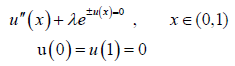(1)

The closed form for the exact solution to eqn. (1) is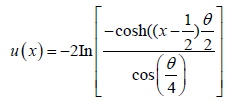(2)

where θ satisfies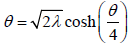. Eqn. (1) has zero, one or two solutions when λ>λc;λ=λc and λ<λc respectively, where the critical value λc satisfies the following equation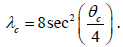It was evaluated from that the critical value of λc has a value of λc=3.513830719.

This type of equation has many applications including the modelling of a combustion in a numerical slab, the ignition of fuel of the thermal theory, the thermal reaction process modelling, the expansion of the universe model and open questions regarding this theory, chemical reaction theory and nanotechnology .

There has been numerous analytic and numerical methods that has been applied for solving Bratu's equation with different forms of exact solutions [2,3]. For instance, B-spline method , parametric spline , non-polynomial spline , quintic spline , cubic spline [8,9], Sinc-Galerkin [10,11], lie group shooting , Adomian decomposition method [13-17], Homotopy perturbation method [18-20], optimal perturbation , successive differentiation method [22,23], Chebychev wavelet [24,25], Legendre wavelet , variation iteration [27-29], iterative finite difference , genetic algorithm based methods , multi step iterative , neural network [33,34], particle swarm shooting  and pseudospectral method [36,37]. In addition to the standard Bratu problem, there are other Bratu-type problems which will be introduced and examined later.

Bernoulli polynomials have gained increasing importance in numerical analysis since they are straightforward and need less computational errors. Many researchers have been working on proving the efficiency of this method [38-43].

The organization of the paper is as follows. We recall the basic concepts of Bernoulli polynomials and their relevant properties needed hereafter. Bernoulli method is presented for solving the general Bratu's type equations. Some numerical examples are presented along with a comparison with other techniques. Finally, the closing stage which provides the conclusions of the study [44-47].

#### Fundamental Relations

Bernoulli polynomials play an important role in different areas of mathematics, including number theory and the theory of finite differences. They are also can be found in the integral representation of the differentiable periodic functions, since they are employed for approximating such functions in term of polynomials. The classical Bernoulli polynomials BN(x) is usually defined by means of exponential generating functions .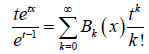from which we can find the following known expansion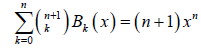which is the most known expansion of the Bernoulli polynomials and from we can generate the first few polynomials as Figure 1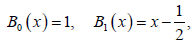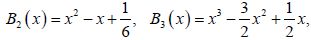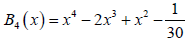these polynomials have many interesting properties from which the following Figure 1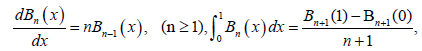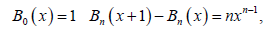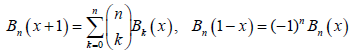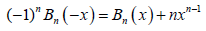In the next we will introduce Bernoulli matrix of differentiation that will be needed later.

Bernoulli operational matrix of differentiation

We will use Bernoulli approximation technique to approximate the solution of eqn. (1) expressed in the truncated Bernoulli series in the form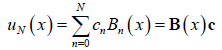(3)

where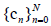are the unknown Bernoulli coe cients, N is any chosen positive integer such that N ≥2, and BN(x),n=0; 1; : : : ; N are the Bernoulli polynomial of the rst kind which are constructed according to equation (2), the Bernoulli coeffcient vector c and the Bernoulli vector B(x) are given by

ct=[c0; c1,…..,cN ]; B(x)=[B0(x); B1(x); : : : ; BN (x)].

According to eqn. (3)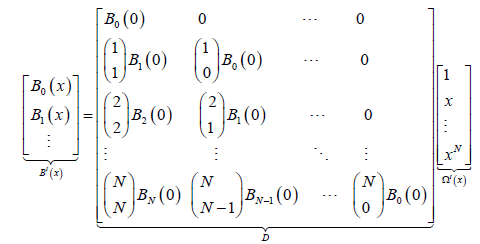Since D is a lower triangular matrix with nonzero diagonal elements and det (D)=1, so D is an invertible matrix. Thus, the Bernoulli vector can be given directly from

B(x)=Ω(x) Dt (4)

note that []t, denotes transpose of the matrix [] and Bt(x) and Ω(x) be the (N+1)×1 and D is the (N+1)×(N+1) operational matrix whose elements are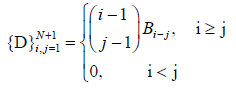(5)

Now, the matrix forms of the solution functions as

yN =Ω(x) Dt c (6)

According to the eqn. (5) the following formula is concluded evidently. Also, the relation between Ω(x) and it's ith derivative Ω(1) (x) is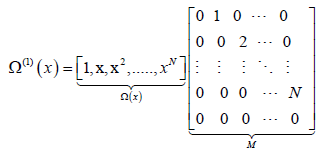(7)

and the following formula holds as

Ω(k)(x)=Ω(x)Mk, k=1,2 (8)

where Ω(i)(x) is denoting the it’s derivative of Ω(x), we have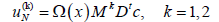(9)

#### Application of the Proposed Method

First, we need to treat the nonlinear term in eqn. (1) by expanding it using Taylor series expansion in the form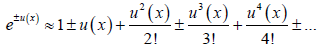(10)

By substituting the expanded term from eqn. (10) into eqn. (1), the equation becomes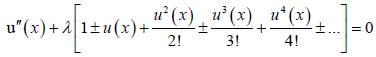(11)

Second, we will use the shifted chebychev defined on the interval [0,1] in the form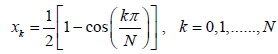After substituting those collocation points into eqn. (11), we reach the following system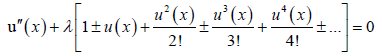(12)

from eqn. (12), we need to approximate the term uv(xk),k =0,1, 2, 3; : : : . We will need the following theorem.

Theorem

The approximation of the function uv(xk), k=0,1,…., N can be represented according to the following relation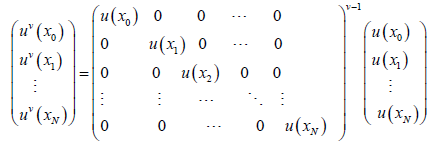(13)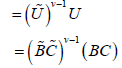Where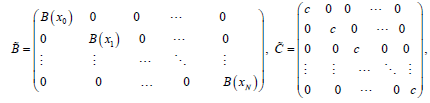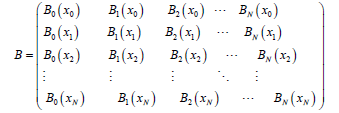By substituting the above theorem into eqn. (12), we reach the following theorem.

Theorem

If the assumed approximate solution of the problem eqn. (12) is eqn. (8), then the discrete Bernoulli system is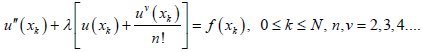(14)

Proof: If we replace each term of (12) with its corresponding approximation given

by eqns.(2), (9) and (13) and substituting x=xk collocation points.

The matrix form for this system is

Θc=F (15)

Where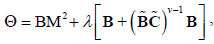And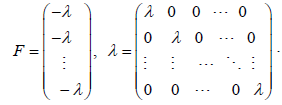The matrix form of the boundary conditions represented in eqn. (1) will be in the form

B(0)=[B0(0),B1(0),B2(0),...,BN(0)]=0

B(1)=[B0(1),B1(1),B2(1),...,BN(1)]=0 (16)

By replacing two rows of the augmented matrix [Θ: F] with the boundary conditions defined from eqn. (16), we have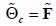(17)

Now we have a nonlinear system of N+1 equation in N+1 unknown coefficient c. We can obtain these coeffcients by solving the above nonlinear system using the following algorithm.

#### Algorithm

1. Input (integer) N.

Input (double) tol.

Input (array) cold=c0, (initial approximation, c0 with N +1 dimension, are chosen so that the boundary conditions are satis ed).

2.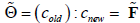is a linear algebraic equation system which is solved and cNew is found.

Go to (2).

2.1 If |Cold-cNew|<tol then cNew=c, break (the program is finished).

2.2 Else then cold←cNew.

3. Go to (2).

#### Numerical Examples

To illustrate the ability, reliability and the performance of the proposed method for Bratu's problem, some examples are provided. The results reveal that the method is very effective and simple. All computations were carried out using Matlab 2014a on a personal computer. The absolute error can be calculated according to the following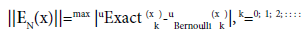Example 1

First, we consider the initial value problem in the form [31,9,25,21,15,46]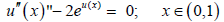subject to the initial conditions

u′(0) = u(0) = 0

which has the exact solution given by

u(x)=−2ln[cos(x).

Table 1 exhibits the maximum absolute error at different values of N along with the elapsed CPU time in seconds. Also, in Table 2 a comparison between the reported results in [31,9,25,21,15,46] along with our method at N=18. This table indicates that our method provide better results than the other methods. Figure 2 demonstrates the Bernoulli approximate solution versus the exact solution for x 2 [0; 1].

N ||EN(x)|| CPU time (sec)
4 1.39189E-02 1.264
6 8.70130E-04 1.329
8 5.39038E-05 1.670
10 3.33058E-06 2.310
12 2.05470E-07 2.868
14 1.26651E-08 3.674
16 7.80110E-10 4.469
18 4.80861E-11 11.98

Table 1: Maximum absolute error and CPU time for Example 4.1.

 Presented method, N=18 4.809e-11 Optimal spline method  3.45e-06 Restarted Adomian decomposition  0.0005745 Optimal perturbation iteration  0.000423 Chebychev wavelet method  1.528e-05 ASM method  6.22e-07 Taylor wavelet  7.801e-08

Table 2: Comparison of maximum absolute error for Example 1.

Example 2

Next, we apply our method for the solution of the special form of Bratu equation [4-12,17,25,28,30,33-34,46]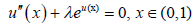subject to the boundary conditions

u(0)=u(1)=0.

with the exact solution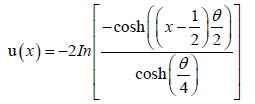(18)

The form of the above equation is very familiar and has a tremendous work for solving it as mentioned in the literature. We applied our method with various (Figure 2) values for=0:1; 0:5; 1; 2; 3 and 3:51. The computed maximum absolute errors at different values of N and are tabulated in Table 3. A comparison with the other methods reported in the literature are presented in Table 4 shows that our method is computationally effective even for=3:51 which is near the critical value. The graph of the approximate solutions for different values of has been plotted in Figure 3.

N λ=0:1 λ=0:5 λ=1:0 λ=2:0 λ=3:0 λ=3:51
4 2.2697E-08 3.4346E-06 3.60159E-05 5.8666E-04 7.4020E-03 2.8391E-02
6 7.2250E-12 5.3149E-09 1.06201E-07 2.8664E-06 5.3078E-05 7.7256E-03
8 3.9100E-15 2.4657E-11 1.12485E-09 8.6920E-08 3.1672E-06 4.1393E-04
10 2.3922E-15 4.7454E-13 1.15237E-11 1.5694E-09 8.8354E-08 9.0424E-06
12 2.3939E-15 4.4039E-13 3.3323E-12 1.2423E-10 4.4392E-09 1.1319E-06
14 - - 3.4237E-12 6.4105E-12 3.1209E-10 7.8326E-08
16 - - - 5.9817E-12 1.2622E-10 7.0247E-09
18 - - - - - 5.5903E-10

Table 3: Maximum absolute error at different λ Example 2.

 Method λ=1:0 λ=2:0 λ=3:51 Presented method, N=14 3.4237E-12 6.4105E-12 5.5903E-10 Sinc galerkin  2.010E-10 1.801E-11 1.4528E-07 Optimal spline  1.810E-07 3.940E-11 × Chebychev wavelet  × 5.487E-06 × Spline method  8.773E-05 8.971E-04 6.890E-06 Lie group shooting  1.018E-06 5.220E-06 7.305E-05 B-spline method  8.892E-06 5.561E-05 1.350E-01 Restarted Adomian  9.410E-07 2.300E-04 × Adomian with Taylor  4.900E-06 6.800E-04 × Spline method  2.178E-06 7.264E-07 × Variational iteration  4.210E-05 1.416E-03 × Parametric spline  5.870E-10 3.530E-08 × Finite di erence  5.703E-10 2.096E-09 6.21E-07 Neural network  3.20E-03 4.95E-03 1.76E-02 Particle swarm shooting  1.150E-08 6.71E-09 3.58E-06 Mexican Hat wavelet  1.90E-08 3.75E-08 1.72E-05 Taylor wavelet  7.760E-12 1.190E-09 1.31E-06 Matlab routine bvp4c  2.47E-07 1.33E-06 1.43E-02 Mathematica routine NDsolve 5.71E-09 5.88E-09 3.01E-07

Table 4: Comparison of maximum absolute error at diﬀerent λ for Example 2.

Figure 3: For λ = 0.5,1.0,2.0,3.0 and λ = 3.51, the approximate solution of the purposed method for N = 14.

Example 3

Now, we turn our attention to the BVP of Bratu's equation in the form [21,31]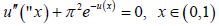subject to the boundary conditions

u(0)=u(1)=0.

This equation is a standard Bratu equation for which λ =π 2 >λc which has two possible solutions in the form u(x)=ln(1±sin( x)). This solution with the negative sign blows up at x=0:5 so we will use the solution of positive sign which is convergent and bounded in the form

u(x)=ln [1+sin( x)]

Maximum absolute error is tabulated in Table 5 along with the CPU time and a comparison is made in Table 6 between our method along with the other methods in [21,31]. From Table 6, we noticed that our method is more accurate than the other existing methods. Figure 4 demonstrates the Bernoulli approximate solution and the exact which appears to be in good agreement with each other.

N kEN(x)k CPU time (sec)
4 2.20480E-03 1.264
6 6.78106E-05 1.329
8 2.64416E-06 1.670
10 1.13839E-07 2.310
12 5.62457E-09 2.868
14 2.64951E-10 3.674
16 4.76705E-11 4.469
18 9.04055E-13 11.98

Table 5: Maximum absolute error and CPU time for Example 3.

 Presented method, N=18 9.04055e-13 Optimal perturbation iteration  8.886e-07 ASM method  8.034e-06 GA-ASM method  3.449e-05 GA method  0.02088

Table 6: Comparison of maximum absolute error for Example 3.

Example 4

Finally, consider another form of Bratu's equation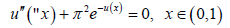subject to the boundary conditions

u(0)=u(1)=0.

with the exact solution

u(x)=-log [1-cos ((0:5+x)π)].

The maximum absolute error for this problem is tabulated in Table 7 at different values of N along with the CPU time. A comparison is made along with other methods which is given in the literature in Table 8 which proves the applicability of the purposed algorithm of providing good results. Figure 5 demonstrates the Bernoulli approximate solution and the exact which appears to be in good agreement with each other.

N ||EN(x)|| CPU time (sec)
4 2.20480E-03 1.065
6 6.78106E-05 1.269
8 2.64416E-06 1.696
10 1.13839E-07 2.266
12 5.62457E-09 2.859
14 2.64951E-10 3.624
16 4.76705E-11 4.794
18 7.46625E-13 5.573

Table 7: Maximum absolute error and CPU time for Example 4.

 Presented method, N=18 7.46625e-13 Sinc-Galerkin  8.39e-09 Optimal Spline method  4.341e-10 GA method  1.293e-06 Taylor Wavelet  1.434e-07

Table 8: Comparison of maximum absolute error for Example 4.

#### Conclusion

In this paper, we showed that Bernoulli-collocation method can be utilized to and an approximate solution of the nonlinear Bratu's type equations. The method reduces the problem into a system of nonlinear algebraic equations and this system is solved using a novel technique. Also, the efficiency of the method with respect to the other method was shown. In comparison to other methods, we illustrated that Bernoullicollocation method has very high accuracy.

#### References

Citation: EL-Gamel M, Adel W, EL-Azab MS (2018) Collocation Method Based on Bernoulli Polynomial and Shifted Chebychev for Solving the Bratu Equation. J Appl Computat Math 7: 407. DOI: 10.4172/2168-9679.1000407

Copyright: © 2018 EL-Gamel M, et al. This is an open-access article distributed under the terms of the Creative Commons Attribution License, which permits unrestricted use, distribution, and reproduction in any medium, provided the original author and source are credited.

Select your language of interest to view the total content in your interested language

##### Recommended Journals
Viewmore
###### Article Usage
• Total views: 747
• [From(publication date): 0-2018 - Aug 18, 2019]
• Breakdown by view type
• HTML page views: 667
• PDF downloads: 80

## Post your commentCan't read the image? click here to refresh
###### Peer Reviewed Journals

Make the best use of Scientific Research and information from our 700 + peer reviewed, Open Access Journals

International Conferences 2019-20

Meet Inspiring Speakers and Experts at our 3000+ Global Annual Meetings

Top